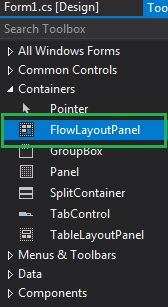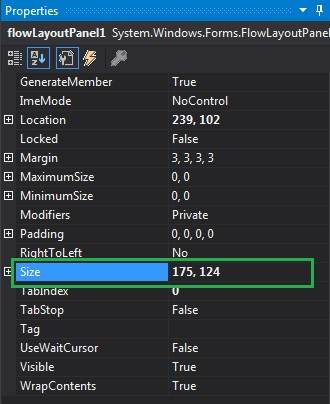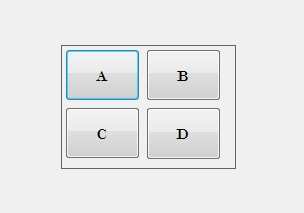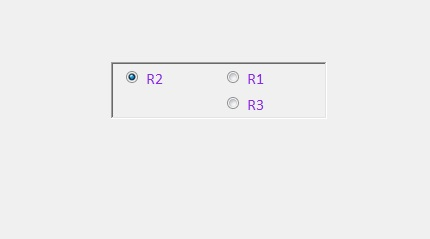Related Articles

# How to set the Size of the FlowLayoutPanel in C#?

• Last Updated : 02 Aug, 2019

In Windows Forms, FlowLayoutPanel control is used to arrange its child controls in a horizontal or vertical flow direction. Or in other words, FlowLayoutPanel is a container which is used to organize different or same types of controls in it either horizontally or vertically. In FlowLayoutPanel control, you can set the size of the control in the form using Size Property. With the help of this property, you can set both the height and width of the control in pixels. You can set this property in two different ways:

1. Design-Time: It is the easiest way to set the size of the FlowLayoutPanel as shown in the following steps:

• Step 1: Create a windows form as shown in the below image:
Visual Studio -> File -> New -> Project -> WindowsFormApp• Step 2: Next, drag and drop the FlowLayoutPanel control from the toolbox to the form as shown in the below image:• Step 3: After drag and drop you will go to the properties of the FlowLayoutPanel and set the size of the FlowLayoutPanel as shown in the below image:Output:2. Run-Time: It is a little bit trickier than the above method. In this method, you can set the size of the FlowLayoutPanel control programmatically with the help of given syntax:

`public System.Drawing.Size Size { get; set; }`

The following steps show how to set the size of the FlowLayoutPanel dynamically:

• Step 1: Create a FlowLayoutPanel using the FlowLayoutPanel() constructor is provided by the FlowLayoutPanel class.
```// Creating a FlowLayoutPanel
FlowLayoutPanel f = new FlowLayoutPanel();
```
• Step 2: After creating FlowLayoutPanel, set the Size property of the FlowLayoutPanel provided by the FlowLayoutPanel class.
```// Setting the size
f.Size = new Size(216, 57);
```
• Step 3: And last add this FlowLayoutPanel control to the form and also adding child controls in the FlowLayoutPanel using the following statements:
```// Adding a FlowLayoutPanel
// control to the form

and

// the FlowLayoutPanel
```

Example:

 `using` `System;``using` `System.Collections.Generic;``using` `System.ComponentModel;``using` `System.Data;``using` `System.Drawing;``using` `System.Linq;``using` `System.Text;``using` `System.Threading.Tasks;``using` `System.Windows.Forms;`` ` `namespace` `WindowsFormsApp50 {`` ` `public` `partial` `class` `Form1 : Form {`` ` `    ``public` `Form1()``    ``{``        ``InitializeComponent();``    ``}`` ` `    ``private` `void` `Form1_Load(``object` `sender, EventArgs e)``    ``{``        ``// Creating and setting the ``        ``// properties of FlowLayoutPanel``        ``FlowLayoutPanel f = ``new` `FlowLayoutPanel();``        ``f.Location = ``new` `Point(380, 124);``        ``f.Size = ``new` `Size(216, 57);``        ``f.Name = ``"Mycontainer"``;``        ``f.Font = ``new` `Font(``"Calibri"``, 12);``        ``f.FlowDirection = FlowDirection.RightToLeft;``        ``f.BorderStyle = BorderStyle.Fixed3D;``        ``f.ForeColor = Color.BlueViolet;``        ``f.Visible = ``true``;`` ` `        ``// Adding this control to the form``        ``this``.Controls.Add(f);`` ` `        ``// Creating and setting the ``        ``// properties of radio buttons``        ``RadioButton r1 = ``new` `RadioButton();``        ``r1.Location = ``new` `Point(3, 3);``        ``r1.Size = ``new` `Size(95, 20);``        ``r1.Text = ``"R1"``;`` ` `        ``// Adding this control ``        ``// to the FlowLayoutPanel``        ``f.Controls.Add(r1);`` ` `        ``RadioButton r2 = ``new` `RadioButton();``        ``r2.Location = ``new` `Point(94, 3);``        ``r2.Size = ``new` `Size(95, 20);``        ``r2.Text = ``"R2"``;`` ` `        ``// Adding this control to``        ``// the FlowLayoutPanel``        ``f.Controls.Add(r2);`` ` `        ``RadioButton r3 = ``new` `RadioButton();``        ``r3.Location = ``new` `Point(3, 26);``        ``r3.Size = ``new` `Size(95, 20);``        ``r3.Text = ``"R3"``;`` ` `        ``// Adding this control to``        ``// the FlowLayoutPanel``        ``f.Controls.Add(r3);``    ``}``}``}`

Output:My Personal Notes arrow_drop_up